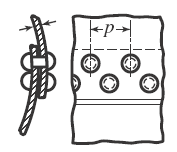Related Resources: calculators

### Double-Riveted Lap-Joint Formulas and Calculator for Stress

Applications and Design
Stress, Deflections and Structural Analysis

Double-Riveted Lap-Joint Formulas and Calculator for Stress and Strength Design.Preview

The efficiency of a riveted joint is equal to the strength of the joint divided by the strength of the un riveted plate, expressed as a percentage.

Resistance to shearing two rivets:

Rs = 2 π d2Ss / 4

Resistance to tearing between two rivets:

Rt = ( p - D) t St

Resistance to crushing in front of two rivets:

Rc = 2 d t Sc

The safe tensile load L based on single shear of the rivets:

L = n Ar Ss

The safe tensile load L based on bearing stress:

L = n Ab Sc

The safe load L based on the tensile stress:

L = Ap St

Efficiency of Design

η = [(safe tensile load L based on single shear of the rivets) / ( l t St) ] 100

Where:

Rs = Resistance to shearing (lbs)
Rt = Resistance to tearing plate between rivets (lbs)
Rc = Resistance to crushing rivet or plate (lbs)
L = Safe Tensile Load (lbs)
η = Strength of Joint / Strength of Un-riveted plate (%)
d = diameter of holes (in)
t = thickness of plate (in)
D = Diameter of the rivet (in)
tc = thickness of cover plates (in)
p = pitch of inner row of rivets (in)
P = pitch of outer row of rivets (in)
Ss = shear stress for rivets (lbs/in2)
St = tensile stress for plates (lbs/in2)
Sc = compressive or bearing stress for rivets or plates (lbs/in2)

Related:

Reference Machinery Handbook, 23rd Edition